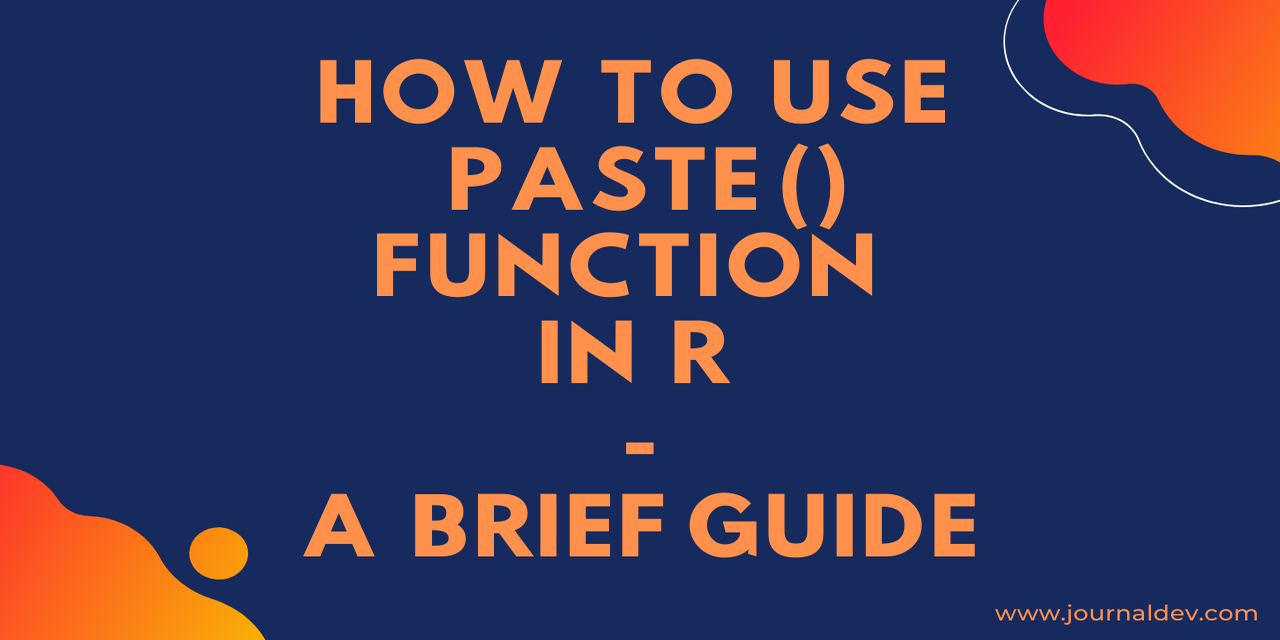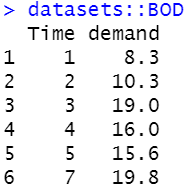# The paste() function in R - A brief guide

Published on August 3, 2022By Prajwal CNWhile we believe that this content benefits our community, we have not yet thoroughly reviewed it. If you have any suggestions for improvements, please let us know by clicking the “report an issue“ button at the bottom of the tutorial.

Using the paste() function in R will be straight and simple. In this tutorial let’s see how we can use paste() to concatenate the strings and values.

paste(): Takes multiple elements from the multiple vectors and concatenates them into a single element.

Along with paste() function, R has another function named paste0(). Yes, you heard it right.

paste0(): The paste0() function has space as its default separator and limits your opportunities in the output as well.

The syntax of the paste() function is,

``````paste(x,sep=" ", collapse=NULL)
``````

Here:

• x = vector having values.
• sep = separator symbols that can be used to separate the elements.
• collapse = It gives a value to collapse.

The syntax of the paste0() function is,

``````paste(x,collapse=NULL)
``````

Where,

• x = vector having the values.
• collapse = It gives a value to collapse.

## How to use the paste() function in R?

A simple paste() will take multiple elements as inputs and concatenate those inputs into a single string. The elements will be separated by a space as the default option. But you can also change the separator value using the ‘sep’ parameter.

``````paste(1,'two',3,'four',5,'six')
``````

Output = “1 two 3 four 5 six”

### Using paste() with a separator argument

The separator parameter in the paste() function will deal with the value or the symbols which are used to separate the elements, which is taken as input by the paste() function.

``````paste(1,'two',3,'four',5,'six',sep = "_")
``````

Output = “1_two_3_four_5_six”

``````paste(1,'two',3,'four',5,'six',sep = "&")
``````

Output = “1&two&3&four&5&six”

### The paste() function with collapse argument

When you pass a paste argument to a vector, the separator parameter will not work. Hence here comes the collapse parameter, which is highly useful when you are dealing with the vectors. It represents the symbol or values which separate the elements in the vector.

``````paste(c(1,2,3,4,5,6,7,8),collapse = "_")
``````

Output = “1_2_3_4_5_6_7_8”

``````paste(c('Rita','Sam','John','Jat','Cook','Reaper'),collapse = ' and ')
``````

Output = “Rita and Sam and John and Jat and Cook and Reaper”

### The paste() function with both separator and collapse arguments

Let’s see how separator and collapse arguments will work. The separator will deal with the values which are to be placed in between the set of elements and the collapse argument will make use of specific value to concatenate the elements into single -string.

``````paste(c('a','b'),1:10,sep = '_',collapse = ' and ')
``````

Output = "a_1 and b_2 and a_3 and b_4 and a_5 and b_6 and a_7 and b_8 and a_9 and b_1

``````paste(c('John','Ray'),1:5,sep = '=',collapse = ' and ')
``````

Output = “John=1 and Ray=2 and John=3 and Ray=4 and John=5”

## How to use paste0() function in R

Paste0() function acts just like paste function but with a default separator.

Let’s see how paste0() function works.

``````paste0('df',1:6)
``````

Output = “df1” “df2” “df3” “df4” “df5” “df6”

You can see that the paste0() function has the default separator value. Now let’s see how paste0() function works with the collapse parameter.

### Using the paste0() function with collapse argument

The collapse argument in the paste0() function is the character, symbol, or a value used to separate the elements.

``````paste0('df',1:5,collapse = '_')
``````

Output = “df1_df2_df3_df4_df5”

``````paste0('df',1:5,collapse = ' > ')
``````

Output = “df1 > df2 > df3 > df4 > df5”

As you may observe the above results, the paste0() function returns a string with a default separator and a specified collapse argument as well.

## How to use paste() function in a data frame in R

You can also use the paste() function to paste the values or elements present in a data frame.

Let’s see how it works with the ‘BOD’ data set.``````datasets::BOD
paste(BOD\$Time,sep = ',',collapse = '_')
``````

Output = “1_2_3_4_5_7”

``````datasets::BOD
paste(BOD\$demand,sep = ',',collapse = '_')
``````

Output = “8.3_10.3_19_16_15.6_19.8”

## Conclusion

R offers numerous functions to make your analysis simpler but efficient. Among them the paste() function is very useful in concatenating the strings and the elements into a single string as well.

In this tutorial we have gone through various aspects of the paste() and paste0() functions. Both these will be really helpful in data analysis.

That’s all for now. Stay tuned for more R tutorials. Happy pasting!!!

More study:

Thanks for learning with the DigitalOcean Community. Check out our offerings for compute, storage, networking, and managed databases.Prajwal CN

author

#### Still looking for an answer?

Ask a questionSearch for more help